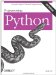# Section 20.9. Sorting Sequences

### 20.9. Sorting Sequences

Another staple of many systems is sorting: ordering items in a collection according to some constraint. The script in Example 20-24 defines a simple sort routine in Python, which orders a list of objects on a field. Because Python indexing is generic, the field can be an index or a keythis function can sort lists of either sequences or mappings.

##### Example 20-24. PP3E\Dstruct\Classics\sort1.py

 ` def sort(list, field): res = [] # always returns a list for x in list: i = 0 for y in res: if x[field] <= y[field]: break # list node goes here? i = i+1 res[i:i] = [x] # insert in result slot return res if _ _name_ _ == '_ _main_ _': table = [ {'name':'john', 'age':25}, {'name':'doe', 'age':32} ] print sort(table, 'name') print sort(table, 'age') table = [ ('john', 25), ('doe', 32) ] print sort(table, 0) print sort(table, 1) `

Here is this module's self-test code in action:

` C:\...\PP3E\Dstruct\Classics>python sort1.py [{'age': 32, 'name': 'doe'}, {'age': 25, 'name': 'john'}] [{'age': 25, 'name': 'john'}, {'age': 32, 'name': 'doe'}] [('doe', 32), ('john', 25)] [('john', 25), ('doe', 32)] `

Since functions can be passed in like any other object, we can easily allow for an optional comparison function. In the next version (Example 20-25), the second argument takes a function that should return TRue if its first argument should be placed before its second. A lambda is used to provide an ascending-order test by default. This sorter also returns a new sequence that is the same type as the sequence passed in, by applying the slicing techniques used in earlier sections: if you sort a tuple of nodes, you get back a tuple.

##### Example 20-25. PP3E\Dstruct\Classics\sort2.py

 ` def sort(seq, func=(lambda x,y: x <= y)): # default: ascending res = seq[:0] # return seq's type for j in range(len(seq)): i = 0 for y in res: if func(seq[j], y): break i = i+1 res = res[:i] + seq[j:j+1] + res[i:] # seq can be immutable return res if _ _name_ _ == '_ _main_ _': table = ({'name':'doe'}, {'name':'john'}) print sort(list(table), (lambda x, y: x['name'] > y['name'])) print sort(tuple(table), (lambda x, y: x['name'] <= y['name'])) print sort('axbyzc') `

This time, the table entries are ordered per a field comparison function passed in:

` C:\...\PP3E\Dstruct\Classics>python sort2.py [{'name': 'john'}, {'name': 'doe'}] ({'name': 'doe'}, {'name': 'john'}) abcxyz `

This version also dispenses with the notion of a field altogether and lets the passed-in function handle indexing if needed. That makes this version much more general; for instance, it's also useful for sorting strings.Programming Python
ISBN: 0596009259
EAN: 2147483647
Year: 2004
Pages: 270
Authors: Mark Lutz